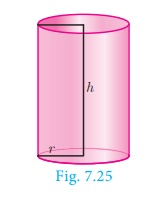Home | | Maths 10th Std | Volume of a solid right circular cylinder

# Volume of a solid right circular cylinder

The volume of a right circular cylinder of base radius ‘r’ and height ‘h’ is given by V = (Base Area) × (Height)

Volume

Having discussed about the surface areas of cylinder, cone, sphere, hemisphere and frustum, we shall now discuss about the volumes of these solids.

Volume refers to the amount of space occupied by an object. The volume is measured in cubic units.

Volume of a solid right circular cylinder

The volume of a right circular cylinder of base radius ‘r’ and height ‘h’ is given by V = (Base Area) × (Height) = πr2 × h = πr2h cubic units.

Therefore, Volume of a cylinder = πr2h cu. Units.Tags : Formula | Mensuration | Mathematics , 10th Mathematics : UNIT 7 : Mensuration
Study Material, Lecturing Notes, Assignment, Reference, Wiki description explanation, brief detail
10th Mathematics : UNIT 7 : Mensuration : Volume of a solid right circular cylinder | Formula | Mensuration | Mathematics